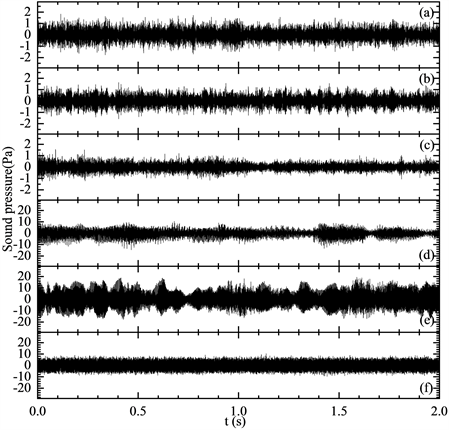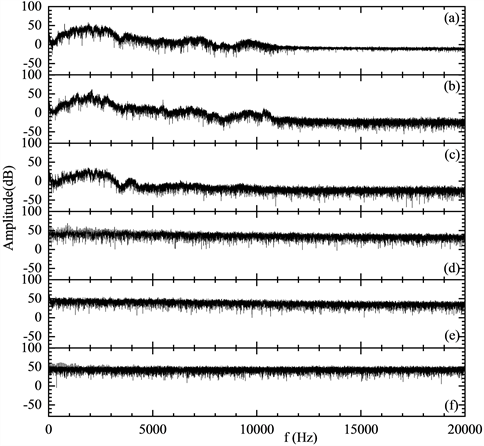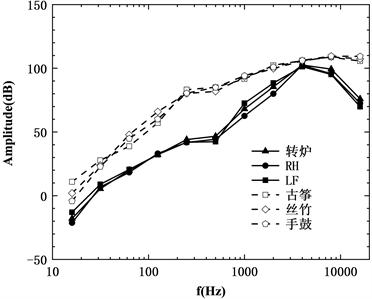#### 期刊菜单

Analysis of Characteristics of Noise in Steelmaking Factory
DOI: 10.12677/MEng.2022.93031, PDF , HTML, XML, 下载: 148  浏览: 227  国家自然科学基金支持

Abstract: In order to describe the characteristics of the noise in a steel-making plant accurately, the noise of the typical metallurgical reactors in a steel-making plant was measured by the professional microphone data acquisition system and VA-Lab2 software, and the noise spectrum, total sound level, box-counting dimension, variational dimension and information dimension of different metallurgical reactors in steel plant were analyzed by home-made source codes. The results show that, on the base of A-weighted sound level, convector, RH furnace and ladle furnace have a similar rule on the sound octave, Guzheng, Jiangnan traditional stringed and woodwind instruments and tabor have a similar rule on the sound octave, and the sound octave of musical instruments is greater than that of metallurgical reactors. The noise box dimension converter, ladle furnace and RH furnace are 1.01, the variational dimension is 1.31, and the information dimension is 4.1. The fractal dimension (box-counting dimension and variational dimension) of the sound signal in the time domain can differentiate between the noise in the steelmaking plant and the music, but the information dimension of the sound frequency domain signal can’t differentiate between the noise in the steelmaking plant and the music.

1. 引言

1984年，周浩庆  首次报道了钢铁企业炼铁、炼钢、轧钢等生产单位的声压级，引入了钢铁厂噪声主动控制的技术思想。2003年，Mizoue  最先分析了日本钢铁厂男性工人吸烟、噪声频率、年龄、听力受损之间的关系，为钢铁厂噪声评价奠定了基础。本文利用专用音频数据采集设备采集并记录炼钢厂主要冶金设备(转炉、LF炉和RH炉)的有效噪声信号；接着利用VA-Lab2软件的数据分析模块以文本形式导出所需噪声的时域数据，最后采用基于Fortran语言开发的自主产权软件Noise Analysis 1.0分析炼钢厂冶金设备工作噪声的特点。为了更加明确地表征炼钢厂的噪声特点，古筝乐曲(山不转水转)、江南丝竹乐曲(夜行船)和手鼓乐曲(乌兰巴托的夜)被选为对比样本。本文涉及的具体分析方法如下：首先利用快速傅立叶变换得到声信号的频谱，然后引进电磁信号的盒维数方法和变分估计方法获取声音时域信号的分形维数，最后利用电磁信号的信息维数的计算方法得到声音频域信号的信息维数。

2. 噪声分析方法

2.1. 采样

2.2. 时域信号

2.2.1. A计权声压级

1) 基于参考声压pref，利用声压级数据计算序号为i的中心频率声压级Li

${L}_{i}=20\mathrm{lg}\frac{\underset{j=1}{\overset{m}{\sum }}{p}_{ij}}{{p}_{\text{ref}}}$ (1)

2) 基于序号为i的中心频率声压级Li评估A计权总声压级L'。

${L}^{\prime }=10\mathrm{lg}\left(\underset{i=1}{\overset{n}{\sum }}{10}^{\frac{A+{L}_{i}}{10}}\right)$ (2)

2.2.2. 盒维数

${\delta }_{1}=\underset{i=1}{\overset{n}{\sum }}|{p}_{i}-{p}_{i+1}|$ (3)

${\delta }_{2}=\underset{i=1}{\overset{n/2}{\sum }}\left[\mathrm{max}\left({p}_{2i-1},{p}_{2i},{p}_{2i+1}\right)-\mathrm{min}\left({p}_{2i-1},{p}_{2i},{p}_{2i+1}\right)\right]$ (4)

${D}_{b}=1+{\mathrm{log}}_{2}\frac{{\delta }_{1}}{{\delta }_{2}}$ (5)

2.2.3. 变分估计分形维数

$OS{C}_{\epsilon }\left(p,\tau \right)=|\underset{|\tau -t|<\epsilon }{\mathrm{max}}\left(p\left(t\right)\right)-\underset{|\tau -t|<\epsilon }{\mathrm{min}}\left(p\left(t\right)\right)|$ (6)

$VA{R}_{\epsilon }\left(p,{t}_{1},{t}_{2}\right)=\frac{1}{{t}_{2}-{t}_{1}}{\int }_{{t}_{1}}^{{t}_{2}}OS{C}_{\epsilon }\left(p,\tau \right)\text{d}\tau$ (7)

${D}_{V}=\underset{\epsilon \to 0}{\mathrm{lim}}\left(2-\frac{\mathrm{lg}VA{R}_{\epsilon }\left(f,{t}_{1},{t}_{2}\right)}{\mathrm{lg}\epsilon }\right)$ (8)

2.3. 频域信号

${P}_{j}=\frac{N{\left(F\right)}_{j}}{N\left(F\cap {A}_{j}\right)}\text{ }j=1,2,\cdots ,N$ (9)

${S}_{I}=\underset{j=1}{\overset{N}{\sum }}{P}_{j}\mathrm{lg}\frac{1}{{P}_{j}}$ (10)

${D}_{I}=\underset{\epsilon \to 0}{\mathrm{lim}}\frac{{S}_{I}\left(\epsilon \right)}{\mathrm{lg}\epsilon }$ (11)

3. 炼钢厂噪音采集及特性分析

3.1. 炼钢设备的时域特征(a) 转炉；(b) RH炉；(c) LF炉；(d) 古筝；(e) 江南丝竹；(f) 手鼓

Figure 1. Time-domain signal of sound in steel-making equipments and musical instrument

3.2. 炼钢设备的频谱特征(a) 转炉；(b) RH炉；(c) LF炉；(d) 古筝；(e) 江南丝竹；(f) 手鼓

Figure 2. Noise spectrum of steel-making equipments and musical instrumentFigure 3. A-weighted octave of the sound

3.3. 炼钢设备噪声现状Table 1. Total sound pressure level of steel-making equipments on the base of A-weighted sound level

3.4. 炼钢设备的分形维数和信息维数Table 2. Fractal dimension and information dimension of noise for steel-making equipments

4. 结论

1) 炼钢厂噪音的特点是强扰动的随机出现，振幅的切换整体上呈现随机无节奏。音乐时域信号可能出现等幅振荡，也可能出现纺锤形，强弱切换的时间量级为0.1秒。

2) 不同炼钢设备(转炉、LF炉、RH炉)噪声在A计权下声压的倍频程变化规律相似；乐器(古筝、江南丝竹和手鼓)乐声在A计权下声压的倍频程变化规律相似。乐器乐声的倍频程高于炼钢设备噪声。

3) 不同炼钢设备(转炉、LF炉、RH炉)的噪音具有不同频率的音强和音高变化混乱、音量过大的特点；乐器(古筝、江南丝竹和手鼓)乐声的特点是不同频率音高和音强变化平缓的特点。

4) 炼钢设备(转炉、LF炉、RH炉)噪声的盒维数约为1.01，变分估计分形维数约为1.31，信息维数约为4.1。

5) 声音时域信号的分形维数(盒维数和变分估计分形维数)能区分炼钢厂噪声和乐声，而声音频域信号的分形维数则无法区分炼钢厂噪声和乐声。

6) 通过对钢厂不同炼钢设备噪音特性分析及与不同乐器乐声特性对比，为钢厂治理噪音提供参考依据。

  戚作秋, 王宏, 常文文, 王翘秀. 生产性噪声对工作人员脑认知影响的ERP分析[J]. 东北大学学报(自然科学版), 2017, 38(11): 1590-1594.  Chung, I., Chu, I.M. and Cullen, M.R. (2012) Hearing Effects from Intermittent and Continuous Noise Exposure in a Study of Korean Factory Workers and Firefighters. BMC Public Health, ‏12, Article No. 87. https://doi.org/10.1186/1471-2458-12-87  Zhou, F., Shrestha, A., Mai, S., Tao, Z., Li, J., Wang, Z. and Meng, X. (2019) Relationship between Occupational Noise Exposure and Hypertension: A Cross-Sectional Study in steel Factories. American Journal of Industrial Medicine, 62, 961-968. https://doi.org/10.1002/ajim.23034  Nyarubeli, I.P., Tungu, A.M., Bratveit, M., Sunde, E., Kayumba, A.V. and Moen, B.E. (2018) Variability and Determinants of Occupational Noise Exposure among Iron and Steel Factory Workers in Tanzania. Annals of Work Exposures and Health, 62, 1109-1122. https://doi.org/10.1093/annweh/wxy071  Wu, C., Huang, C. and Chen, H. (2015) Dimensional Emotion Recognition in Shispered Speech Signal Based on Cognitive Performance Evaluation. Journal of Southeast University (English Edition), 31, 311-319  Beriat, G.K. and Ozkan, S. (2012) The Evalution of Central Auditory Processing in the Geriatric Population. Turkish Journal of Geriatrics, 15, 75-80.  Terlecki, R.P. and Triest, J.A. (2007) A Contemporary Evaluation of the Auditory Hazard of Extracorporeal Shock Wave Lithotripsy. Urology, 70, 898-899. https://doi.org/10.1016/j.urology.2007.06.1151  Bigerelle, M. and Iost, A. (2000) Fractal Dimension and Classification of Music. Chaos, Solitons and Fractals, 11, 2179-2192. https://doi.org/10.1016/S0960-0779(99)00137-X  Voss, R.F. and Clarke, J. (1975) ‘1/f Noise’ in Music and Speech. Nature, 258, 317-318. https://doi.org/10.1038/258317a0  Zhao, X., Liu, G., Zhang, C., Xia, D. and Lu, Z. (2018) Fractal Acoustic Metamaterials for Transformer Noise Reduction. Applied Physics Letters, 113, Article ID: 074101. https://doi.org/10.1063/1.5038431  Hamidi, M., Ghassemian, H. and Imani, M. (2018) Classification of Heart Sound Signal Using Curve Fitting and Fractal Dimension. Biomedical Signal Processing and Control, 39, 351-359. https://doi.org/10.1016/j.bspc.2017.08.002  Su, Z. and Wu, T. (2007) Music Walk, Fractal Geometry in Music. Physica A: Statistical Mechanics and Its Applications, 380, 418-428. https://doi.org/10.1016/j.physa.2007.02.079  Rankin, S.K., Fink, P.W. and Large, E.W. (2014) Fractal Structure Enables Temporal Prediction in Music. Journal of the Acoustical Society of America, 136, EL256-EL262. https://doi.org/10.1121/1.4890198  周浩庆. 冶金设备的噪声源与防治[J]. 江苏冶金, 1984(4): 67-69.  Mizoue, T., Miyamoto, T. and Shimizu, T. (2003) Combined Effect of Smoking and Occupational Exposure to Noise on Hearing Loss in Steel Factory Workers. Occupational and Environmental Medicine, 60, 56-59. https://doi.org/10.1136/oem.60.1.56  Liu, Y., Liu, Y., Yuan, H. and Ji, L. (2018) Research on the Correlation between the Frequency-Domain Characteristics of Corona Current and A-Weighted Sound Level of Audible Noise for UHV DC Transmission Line. IET Generation Transmission & Distribution, 12, 2549-2556. https://doi.org/10.1049/iet-gtd.2017.0467  Walker, E.D., Hart, J.E., Koutrakis, P., Cavallari, J.M., VoPham, T., Luna, M., et al. (2017) Spatial and Temporal Determinants of A-Weighted and Frequency Specific Sound Levels—An Elastic Net Approach. Environmental Research, 159, 491-499. https://doi.org/10.1016/j.envres.2017.08.034  Ho, D.S., Lee, C.K., Wang, C.C. and Chuang, M. (2004) Scaling Characteristics in the Taiwan Stock Market. Physica A: Statistical Mechanics and Its Applications, 332, 448-460. https://doi.org/10.1016/j.physa.2003.10.023  Rakhshandehroo, G.R., Shaghaghian, M.R., Keshavarzi, A.R. and Talebbeydokhti, N. (2009) Temporal Variation of Velocity Components in a Turbulent Open Channel Flow: Identi-fication of Fractal Dimensions. Applied Mathematical Modelling, 33, 3815-3824. https://doi.org/10.1016/j.apm.2008.12.009  Dubuc, B. and Dubuc, S. (1996) Error Bounds on the Estimation of Fractal Dimension. SIAM Journal on Numerical Analysis, 33, 602-626. https://doi.org/10.1137/0733032  Duan, S., Wen, T. and Jiang, W. (2019) A New Information Dimension of Complex Network Based on Renyi Entropy. Physica A: Statistical Mechanics and Its Applications, 516, 529-542. https://doi.org/10.1016/j.physa.2018.10.045  Ramirez-Arellanoa, A., Hernandez-Simonb, L.M. and Bo-ry-Reyesb, J. (2020) A Box-Covering Tsallis Information Dimension and Non-Extensive Property of Complex Net-works. Chaos, Solitons & Fractals, 132, Article ID: 109590. https://doi.org/10.1016/j.chaos.2019.109590  Geiger, B.C. and Koch, T. (2019) On the Information Dimension of Stochastic Processes. IEEE Transactions on Information Theory, 65, 6496-6518. https://doi.org/10.1109/TIT.2019.2922186